• 华工信号与系统实验
千次阅读 多人点赞
2020-06-07 11:32:17

信号与系统[实验三–利用DFT分析模拟信号频谱]
实验题目

1. 利用FFT分析信号 的频谱。
(1) 确定DFT计算的各参数（抽样间隔，截短长度，频谱分辨率等）；
(2) 比较理论值与计算值，分析误差原因，提出改善误差的措施。
2. 分析周期信号的频谱时，如果分析长度不为整周期，利用fft函数计算并绘出其频谱，总结对周期信号进行频谱分析时，如何选取信号的分析长度。
3. 假设一实际测得的一段信号的长度为0.4s，其表达式为
x(t)=cos(2pf1t)+0.75 cos(2pf2t)
其中f1=100Hz，f2=110Hz。当利用FFT近似分析该信号的频谱时，需要对信号进行时域抽样。试确定一合适抽样频率fsam，利用DFT分析信号x(t)的频谱。
若在信号截短时使用Hamming窗，由实验确定能够分辨最小谱峰间隔Df和信号长度Tp的关系。若采用不同参数的Kaiser窗，重新确定能够分辨最小谱峰间隔Df和信号长度Tp 的关系。
4. 产生一个淹没在噪声中的信号x(t)，例如由50Hz和120Hz的正弦信号以及一个零均值的随机噪声叠加而成。确定抽样间隔和信号截短长度，分析信号的频谱，指出50Hz和120Hz的正弦成分对应的谱峰位置，详细写出检测信号的步骤和原理。

第一题代码:

fsam=50;
Tp=6;
N=512;
T=1/fsam;
t=0:T:Tp;
x=exp(-2*t);
X=T*fft(x,N);        %频域幅度  去归一化,乘以采样间隔T

subplot(3,1,1);
plot(t,x);
xlabel('t');
ylabel('|X(jw)|')
title('时域波形');

w=(-N/2:N/2-1)*(2*pi/N)*fsam;    %频域频率  去归一化,除以T
y=1./(j*w+2);

subplot(3,1,2);
plot(w,abs(fftshift(X)),w,abs(y),'r-.');
title('幅度谱');
xlabel('w');
ylabel('y=|1/(j*w+1)|')
legend('理论值','计算值');
axis([-10,10,0,1.4])

subplot(3,1,3);
error = abs(abs(fftshift(X))-abs(y));
plot(w,error)
title('理论与计算的误差');


1)抽样间隔 0.02
截短长度 6
频谱分辨率 50/512
2)由图3-1-1可以看出理论值与实际值相差不多.误差原因是采样间隔不够小.
改进:减小采样间隔

第二题代码:

close all;
%题二 ---连续周期函数
T0=1;       %w0 = 2*pi,  T0 = 1  [w0 = 最大公约数]
T1 = 1.5;   %分析长度不为整周期

w0 = 2*pi ; %最大公约数
p = 9; %最高谐次  110/10
N = 99;  %N>2*p+1
T = T0/N;     %周期T0、FFT的点数N、抽样间隔T
T2 = T1/N;

t = 0:T:T0;
t1 = 0:T2:T1;

x=cos(2*pi*5*t)+2*sin(2*pi*9*t);  %周期信号
x1=cos(2*pi*5*t1)+2*sin(2*pi*9*t1);

figure
subplot(2,1,1)
Xm=fft(x,N) /N;  %频域幅度  得到的是傅里叶级数
w=(-(N-1)/2:(N-1)/2)*(2*pi/T0);   %T0为1个周期,即显示-pi  ~  pi
stem(w,abs(fftshift(Xm)));
ylabel('magnitude');
title('分析长度为整周期');

subplot(2,1,2)
Xm1=fft(x1,N)/N;  %利用FFT计算其频谱
w=(-(N-1)/2:(N-1)/2)*(2*pi/T1);
stem(w,abs(fftshift(Xm1)));
ylabel('magnitude');
title('分析长度为非整周期');


第三题代码:

close all;
clear all;

%连续非周期
fsam = 220; %抽样频率,cos经过傅里叶变换相当于频率搬移,f2搬移到-110  +110,为脉冲信号
%fsam = 2*fm
T0 = 0.4;
f01 = 100;
f02 = 110;

w0 = 10*pi ; %最大公约数
p = 11; %最高谐次  110/10

%T=1/fsam;     %周期T0、FFT的点数N、抽样间隔T
T = 0.004;
t=0:T:(T0-T);
N = length(t);
x=cos(2*pi*f01*t)+ 0.75*cos(2*pi*f02*t);

%问1-------------------------------------
figure
subplot(2,1,1)
plot(t,x)
xlabel('t');
title('x(t)');

subplot(2,1,2)
Xm=fft(x,N)/N;  %傅里叶级数
w=(-N/2:N/2-1)*(2*pi/N)/T0;
f = ((-N/2 + 1):N/2)/T0;
stem(f,abs(fftshift(Xm)));
xlabel('f(hz)');
ylabel('magnitude');

%问2-------------------------------------
%在信号截短时使用Hamming窗，由实验确定能够分辨最小谱峰间隔Df和信号长度Tp的关系

%实验验证DF与Tp无关,无论Tp取何值都是相差10HZ,前提是Tp至少有一个周期长度
T2 = 0.004;
T02 = 0.4;
t2 = 0:T2:(T02-T2);
N2 = length(t2)
k2 = 1:N2;

Ham2 = 1/2.*(1-cos(2*pi* k2 /(N2-1)));
x2 = (cos(2*pi*f01*t2)+ 0.75*cos(2*pi*f02*t2)).*Ham2;

figure
subplot(2,1,1)
plot(t2,x2,t2,Ham2,'r-')
legend('x(t)','Ham')
xlabel('t');
title('x(t) and Hamming窗');

subplot(2,1,2)
Xm2=fft(x2,N2)/N2;  %傅里叶级数

f2 = ((-N2/2 + 1):N2/2)/T02;
stem(f2,abs(fftshift(Xm2)));
xlabel('f(hz)');
ylabel('magnitude');

%问3-------------------------------------
%在信号截短时使用Kaiser窗，由实验确定能够分辨最小谱峰间隔Df和信号长度Tp的关系
%实验验证DF与Tp无关,无论Tp取何值都是相差10HZ,前提是Tp至少有一个周期长度
T3 = 0.004;
T03 = 0.4;
t3 = 0:T3:(T03-T3);
N3 = length(t3);
k3 = 1:N3;

beta = 10;
Kai=kaiser(N3,beta);

x3 = (cos((2*pi*f01).*t3)+ 0.75*cos((2*pi*f02).*t3)).*Kai';

figure
subplot(2,1,1)
plot(t3,x3,t3,Kai)

legend('x(t)','Kaiser')
xlabel('t');
title('x(t) and Kaiser窗');
ylabel('x(t)')

subplot(2,1,2)
Xm3=fft(x3,N3)/N3;  %傅里叶级数

f3 = ((-N3/2 + 1):N3/2)/T03;
stem(f3,abs(fftshift(Xm3)));
xlabel('f(hz)');
ylabel('magnitude');



理论上fsam应取220,可是选择220分析的时候,采样间隔为无限循环小数,导致fft后会丢失110Hz的脉冲,所以选择为fsam = 250
无论是Hamming窗还是Kaiser窗,通过实验改变分析长度Tp,得到的结果都是,两个谱峰间的间隔都是10Hz.故能够分辨最小谱峰间隔Df与信号长度Tp 无关.

第四题代码:

% 题4
close all;
clear all;

fsam = 250;
T0 = 1;
T = 1/fsam;
t = 0:T:(T0-T);
N=length(t);
f1 = 50;
f2 = 120;

x = sin(2*pi*f1*t) + sin(2*pi*f2*t) + randn(size(t));

subplot(211)
plot(t,x)
xlabel('t');
ylabel('x(t)');
title('含随机干扰的信号x(t)');

X=T*fft(x,N);        %频域幅度  去归一化,乘以采样间隔T
f=(-(N-1)/2:(N-1)/2)/T0;    %频域频率  去归一化,除以T

subplot(212)
plot(f,abs(fftshift(X)));
title('幅度谱');
xlabel('f(Hz)');
ylabel('magnitude');


由于f1 = 50Hz, f2 = 120Hz,所以fsam = 240Hz
可是如果取fsam = 240Hz,采样间隔为无限循环小数,所以选取为250Hz,

抽样间隔 = 1/250 =0.004 s
信号截短长度 1s
50Hz和120Hz的频谱可在图3-4-1看出
检测信号的步骤和原理:
由于x(t)是混频相加的函数,可通过傅里叶变换得到各频率上的脉冲信号,即可检测出.
本题多了干扰函数,但是干扰函数的幅度较小,不会对在50Hz和120Hz的脉冲信号有太大的影响,所以可以分辨出.matlab
更多相关内容
• 离散时间信号的增采样减采样如何影响离散时间傅里叶变换(DTFT): a):本练习的大多数题都用下面两个信号的有限段进行 b):用解析法计算.. c):定义将信号x[n]扩展K倍... 代码仅做参考,不能确保正确
• 直接给出实验要求: 题目一 %第1题 %题(1) subplot(2,2,1); t1 = -1:0.01:5; u1 = rectpuls(t1-3,4); x1 = -2 * u1; plot(t1,x1) axis([-1 5 -3 1]); title('x(t) = -2u(t-1)'); xlabel('t'); %题(2) subplot(2,2,...

直接给出实验要求: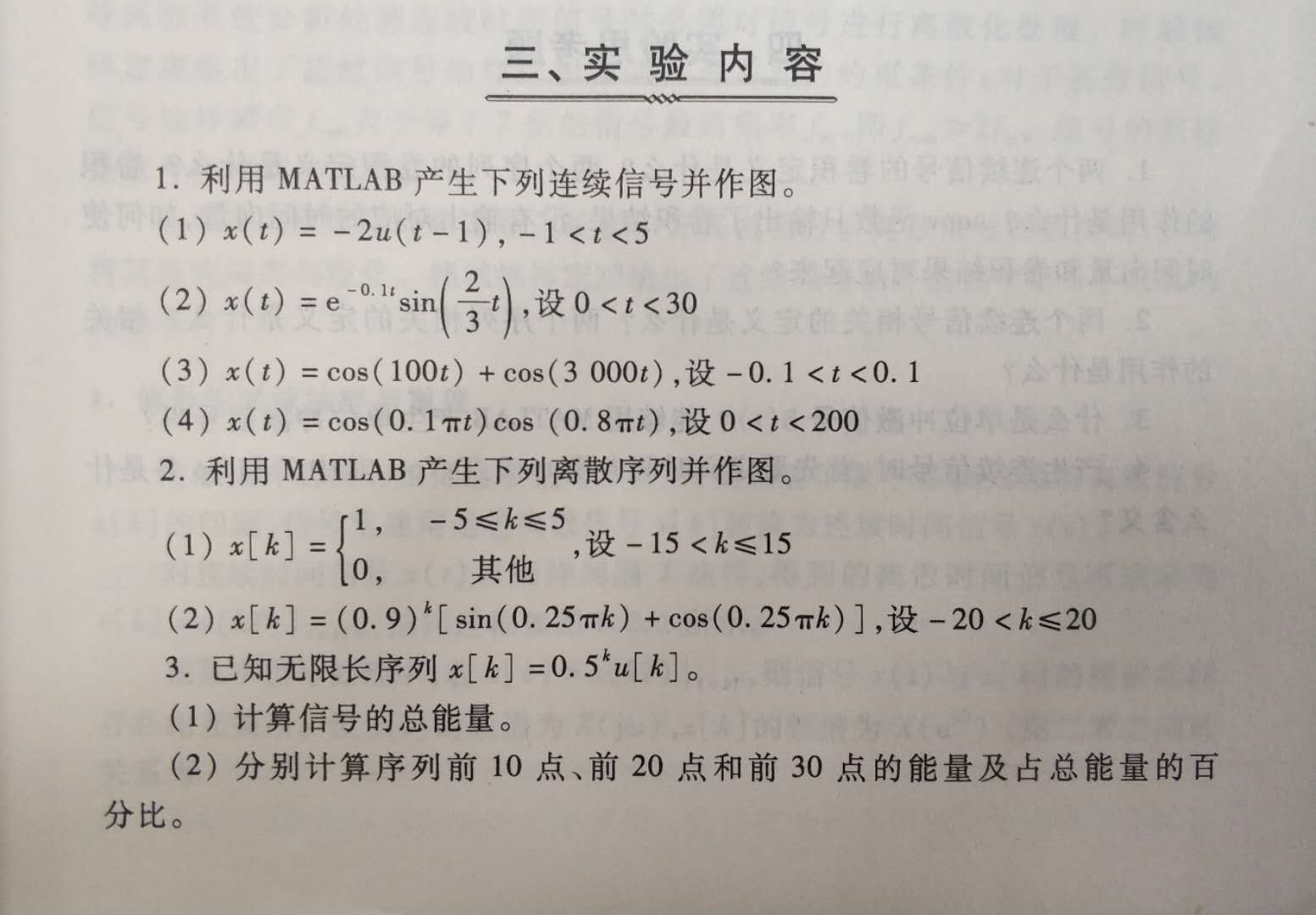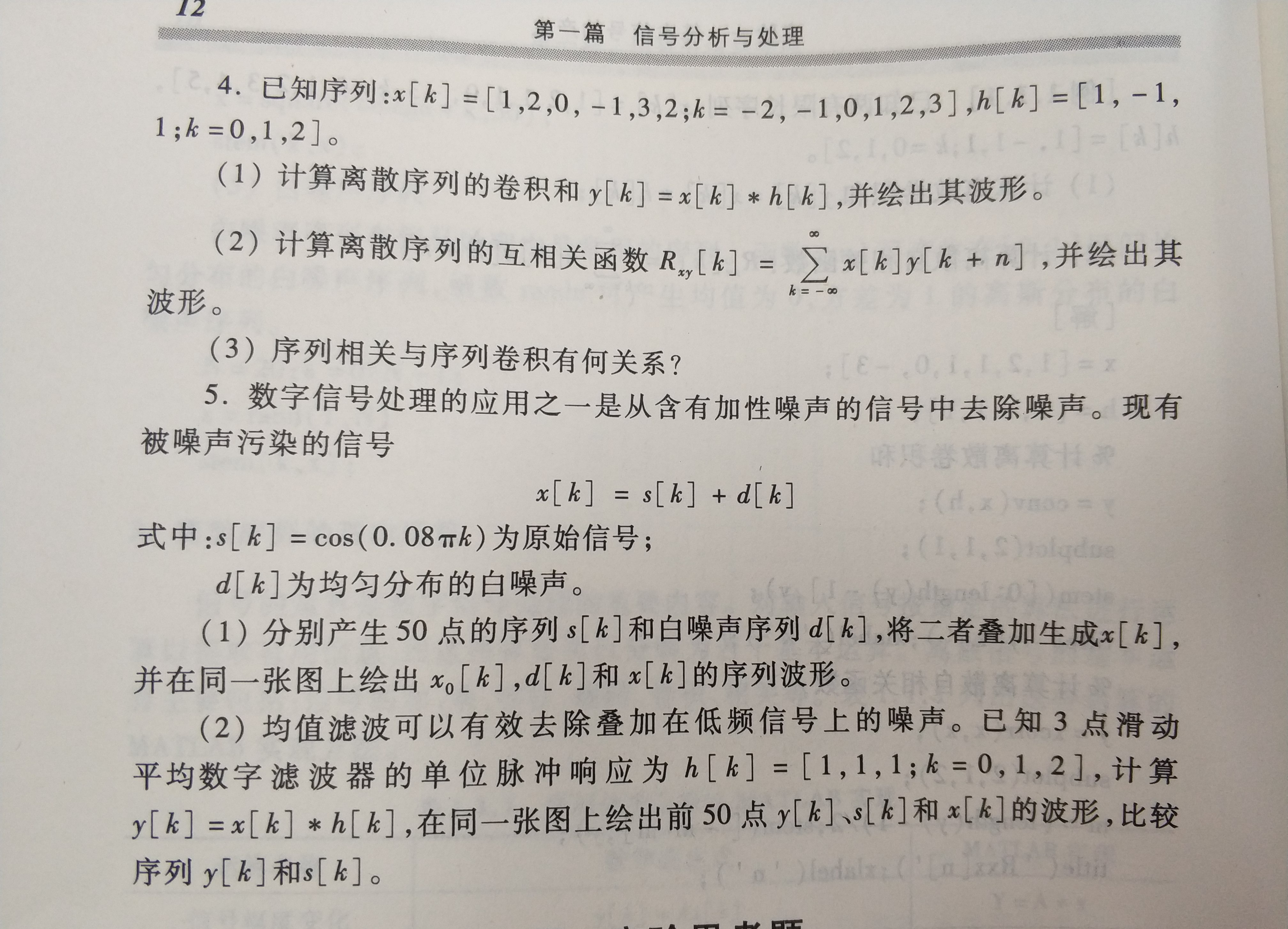题目一

%第1题
%题(1)
subplot(2,2,1);
t1 = -1:0.01:5;
u1 = rectpuls(t1-3,4);
x1 = -2 * u1;
plot(t1,x1)
axis([-1 5 -3 1]);
title('x(t) = -2u(t-1)');
xlabel('t');

%题(2)
subplot(2,2,2);
t2 = 0:0.1:30;
x2 = sin(2*t2/3) .* exp(-0.1*t2);
plot(t2,x2)
xlabel('t');
title('exp(-0.1t)* sin(2t/3)')

%题(3)
subplot(2,2,3);
t3 = -0.1:0.01:0.1;
x3 = cos(100*t3) + cos(3000*t3);
plot(t3,x3)
xlabel('t');
title('cos(100t) + cos(3000t)');

%题(4)
subplot(2,2,4);
t4 = 0:5:200;
x4 = cos(0.1*pi*t4).*cos(0.8*pi*t4);
plot(t4,x4);
xlabel('t');
axis([0 200 -2 2])
title('cos(0.1\pit)cos(0.8\pit)')


题目二

%第2题

%题(1)
subplot(2,1,1);
k1 = -14:15;
x1 = [zeros(1,9), ones(1,11), zeros(1,10)];
stem(k1,x1);
xlabel('k')
title('x[k]');

%题(2)
subplot(2,1,2);
k2 = -19:20;
x2 =(0.9).^k2.*[sin(0.25*pi*k2)+cos(0.25*pi*k2)];
stem(k2,x2);
xlabel('k');
title('x[k]=(0.9)^k[sin(0.25\pik)+cos(0.25\pik)]')


题目三

%第3题
%题(1)
format long
k=-100:1e6;
u = (k>=0);
x = (0.5).^k.*u;
E = sum(abs(x).^2)

%题(2)
k1 = -100:10;
k2 = -100:20;
k3 = -100:30;
u1 = (k1>=0);
u2 = (k2>=0);
u3 = (k3>=0);
x1 = (0.5).^k1.*u1;
x2 = (0.5).^k2.*u2;
x3 = (0.5).^k3.*u3;
E1 = sum(abs(x1).^2)
E2 = sum(abs(x2).^2)
E3 = sum(abs(x3).^2)
p1 = E1/E;
p2 = E2/E;
p3 = E3/E;
fprintf('p1 = %.16f\n',p1)
fprintf('p2 = %.16f\n',p2)
fprintf('p3 = %.16f\n',p3)



题目四

%第4题
clc;
x = [1 2 0 -1 3 2];
h = [1 -1 1];

%题(1)
subplot(2,1,1)
y = conv(x,h)
t = -2:length(y)-3;
stem(t,y)
title('y[k] = x[k] * h[k]')
xlabel('k')

%题(2)
subplot(2,1,2);
R = xcorr(x,y)
m = (length(R) - 1)/2;
stem([-m:m], R);
xlabel('k');
title('R_xy[k]')



题目五

%第5题
clc;

N = 50;
k = 0:N-1;
s = cos(0.08 * pi * k);
d = rand(1,N);

%题(1)
x = s + d;

figure(1)
subplot(3,1,1);
stem(k,s);
xlabel('k');
title('s[k]')

subplot(3,1,2);
stem(k,d);
xlabel('k');
title('d[k]')

subplot(3,1,3);
stem(k,x);
xlabel('k');
title('x[k]=s[k]+d[k]')

%题(2)
figure(2)
h = [1 1 1];
y = conv(x,h)
subplot(3,1,1);
n = 0:length(y)-1;
stem(n,y)
xlabel('n');
title('y[k]=x[k]*h[k]')

subplot(3,1,2);
stem(k,s)
xlabel('k');
title('s[k]');

subplot(3,1,3);
stem(k,x)
xlabel('k');
title('x[k]');


或许有些地方做错了,欢迎纠错~

展开全文• 华南理工大学信号与系统实验 实验五实验报告 优秀
• 信号与系统[实验二–利用DFT分析离散时间信号的频谱] 利用FFT分析信号 的频谱； (1) 确定DFT计算的参数； (2) 进行理论值计算值比较，讨论信号频谱分析过程中误差原因及改善方法。 利用FFT分析信号的频谱； (1) ...

信号与系统[实验二–利用DFT分析离散时间信号的频谱]

1. 利用FFT分析信号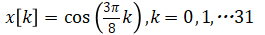的频谱；
(1) 确定DFT计算的参数；
(2) 进行理论值与计算值比较，讨论信号频谱分析过程中误差原因及改善方法。
2. 利用FFT分析信号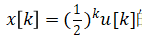的频谱；
(1) 确定DFT计算的参数；
(2) 进行理论值与计算值比较，讨论信号频谱分析过程中误差
原因及改善方法。
3. 有限长脉冲序列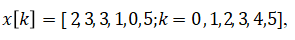, 利用FFT分析其频谱，并绘出其幅度谱与相位谱。
4. 某周期序列由3个频率组成：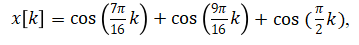, 利用FFT分析其频谱。如何选取FFT的点数N？此3个频率分别对应FFT计算结果X[m]中的哪些点？若选取的N不合适，FFT计算出的频谱X[m]会出现什么情况？
5. 某离散序列,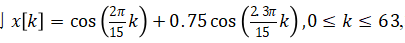利用FFT分析其频谱。
(1) 对x[k]做64点FFT，绘出信号频谱，能分辨出其中的两个频率吗？
(2) 对x[k]补零到256点后计算FFT，能分辨出其中的两个频率吗？
(3) 选用非矩形窗计算FFT，能够分辨出其中的两个频率吗？
(4) 若不能够很好地分辨出其中的两个频谱，应采取哪些措施？
6.已知序列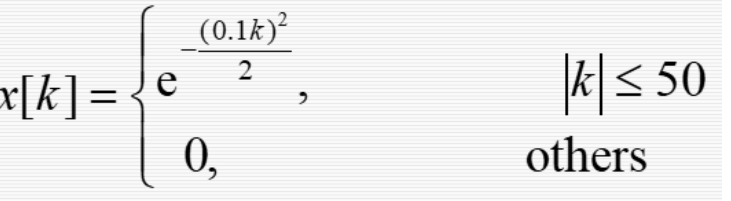利用FFT分析下列信号的幅频特性，频率范围为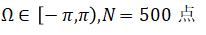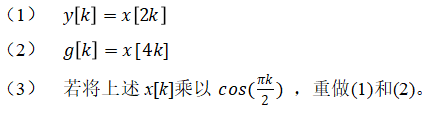第一题代码:
% 题1
close all;
clear all;

N = 32;
N1 = 1000;
k = 0:N-1;
x = cos(3*pi/8*k);
X = fft(x);
Y = abs(fftshift(X));

% x信号散点图
figure
stem(k-N/2,x);
xlabel('k');
ylabel('x=cos(3*pi/8*k)')

% 幅度
figure
subplot(211)
w1 = 2*pi/N;
stem((k-N/2)*w1, Y);
xlabel('Frequency(N=32)');
ylabel('Magnitude');

%相位
subplot(212);
stem((k-N/2)*w1,angle(fftshift(X)));
xlabel('Frequency(N=32)');
ylabel('Phrase');

%改进----------------------------
figure
X2=fft(x,N1);
Y2=fftshift(X2);
w2=[-N1/2:N1/2-1]*(2*pi/N1);
%幅度
subplot(211);
plot(w2,abs(Y2));
xlabel('Frequency(N=1000)');
ylabel('Magnitude');
%相位
subplot(212)
plot(w2,angle(Y2));
ylabel('Phrase');xlabel('Frequency(N=1000)');


第二题代码:

% 题2
close all;
clear all;

N = 20;
N1 = 1000;
k = 0:N-1;
x = (0.5).^k;
X = fft(x);
Y = abs(fftshift(X));

% x信号散点图
figure
stem(k,x);
xlabel('k');
ylabel('x=(1/2)^k')

% |X(e^j^w)|
figure
subplot(211)
w1 = 2*pi/N;
plot((k-N/2)*w1, Y);
xlabel('Frequency(N=20)');
ylabel('Magnitude');

%相位
subplot(212);
plot((k-N/2)*w1,angle(fftshift(X)));
xlabel('Frequency(N=20)');
ylabel('Phrase');

%改进----------------------------
figure
X2=fft(x,N1);
Y2=fftshift(X2);
w2=[-N1/2:N1/2-1]*(2*pi/N1);
%幅度
subplot(211);
plot(w2,abs(Y2));
xlabel('Frequency(N=1000)');
ylabel('Magnitude');
%相位
subplot(212)
plot(w2,angle(Y2));
ylabel('Phrase');xlabel('Frequency(N=1000)');


第三题代码:

close all;
clear all;

% 题3
N = 6;
N1 = 100;
k = 0:N-1;
x = [2,3,3,1,0,5];
X = fft(x);
Y = abs(fftshift(X));

% x信号散点图
figure
stem(k,x);
xlabel('k');
ylabel('x(k)')

%幅度
figure
subplot(211);
w1 = 2*pi/N;
stem((k-N/2)*w1, Y);
xlabel('Frequency(N=6)');
ylabel('Magnitude');

% 相位
subplot(212);
stem((k-N/2)*w1, angle(fftshift(X)));
xlabel('Frequency(N=6)');
ylabel('Phrase');

%改进----------------------------
figure
X2=fft(x,N1);
Y2=fftshift(X2);
w2=[-N1/2:N1/2-1]*(2*pi/N1);
%幅度
subplot(211);
plot(w2,abs(Y2));
xlabel('Frequency(N=100)');
ylabel('Magnitude');
%相位
subplot(212)
plot(w2,angle(Y2));
xlabel('Frequency(N=100)');
ylabel('Phrase');



第四题代码:

close all;
clear all;

% 题4
N = 32;
N1 = 30;
k = 0:N-1;
x = cos(7*pi/16 * k) + cos(9*pi/16 * k) + cos(pi/2 * k);

X = fft(x);
Y = abs(fftshift(X));

% x信号散点图
figure
stem(k,x);
xlabel('k');
ylabel('x(k)')

% 幅度
figure
subplot(211);
w1 = 2*pi/N;
stem((k-N/2)*w1, Y);
xlabel('Frequency(N=32)');
ylabel('Magnitude');

%相位
subplot(212);
stem((k-N/2)*w1,angle(fftshift(X)));
xlabel('Frequency(N=32)');
ylabel('Phrase');

%若N不是32的正整数倍----------------------------
figure
X2=fft(x,N1);
Y2=fftshift(X2);
w2=[-N1/2:N1/2-1]*(2*pi/N1);
%幅度
subplot(211);
stem(w2,abs(Y2));
xlabel('Frequency(N=30)');
ylabel('Magnitude');
%相位
subplot(212)
stem(w2,angle(Y2));
xlabel('Frequency(N=30)');
ylabel('Phrase');


第五题代码:

close all;
clear all;

N = 64;
N1 = 256;
k = 0:N-1;
x = cos(2*pi/15 * k) + 0.75 * cos(2.3*pi/15 * k);

X = fft(x);
Y = abs(fftshift(X));

%问(1)-----------------------------
% x信号散点图
figure
stem(k,x);
xlabel('k');
ylabel('x(k)')

% 幅度
figure
subplot(211);
w1 = 2*pi/N;
stem((k-N/2)*w1, Y);
xlabel('Frequency(N=64)');
ylabel('Magnitude');

%相位
subplot(212);
stem((k-N/2)*w1,angle(fftshift(X)));
xlabel('Frequency(N=64)');
ylabel('Phrase');

%问(2)----------------------------
%若N=256
figure
X2=fft(x,N1);
Y2=fftshift(X2);
w2=[-N1/2:N1/2-1]*(2*pi/N1);
%幅度
subplot(211);
stem(w2,abs(Y2));
xlabel('Frequency(N=256)');
ylabel('Magnitude');
%相位
subplot(212)
stem(w2,angle(Y2));
xlabel('Frequency(N=256)');
ylabel('Phrase');

%问(3)------------------------------
%非矩形窗计算FFT
%汉宁窗+++++++++++++++++++

W = 0.5 - 0.5 * cos(2*pi*k/N);
x3 = x .* W;
X3 = fft(x3);
Y3 = abs(fftshift(X3));

% 幅度
figure
subplot(211);
stem((k-N/2)*w1, Y3);
xlabel('Frequency(N=64)');
ylabel('Magnitude');
title('汉宁窗')
%相位
subplot(212);
stem((k-N/2)*w1,angle(fftshift(X3)));
xlabel('Frequency(N=64)');
ylabel('Phrase');

%高斯窗++++++++++++++
W1 = exp(-0.5 .* (3 * 2*k./(N-1)).^2);
x31 = x .* W1;
X31 = fft(x31);
Y31 = abs(fftshift(X31));

% 幅度
figure
subplot(211);
stem((k-N/2)*w1, Y31);
xlabel('Frequency(N=64)');
ylabel('Magnitude');
title('高斯窗')
%相位
subplot(212);
stem((k-N/2)*w1,angle(fftshift(X31)));
xlabel('Frequency(N=64)');
ylabel('Phrase');

%指数窗+++++++++++++++++++++++
W2 = exp(abs(k-1-N/2)/N);
x32 = x .* W2;
X32 = fft(x32);
Y32 = abs(fftshift(X32));

% 幅度
figure
subplot(211);
stem((k-N/2)*w1, Y32);
xlabel('Frequency(N=64)');
ylabel('Magnitude');
title('指数窗')
%相位
subplot(212);
stem((k-N/2)*w1,angle(fftshift(X32)));
xlabel('Frequency(N=64)');
ylabel('Phrase');

%问(4)----------------------------
%若N=30
N4 = 30;
k4 = 0:N4-1;
x4 = cos(2*pi/15 * k4) + 0.75 * cos(2.3*pi/15 * k4);
figure
X4=fft(x4,N4);
Y4=fftshift(X4);
w4=[-N4/2:N4/2-1]*(2*pi/N4);
%幅度
subplot(211);
stem(w4,abs(Y4));
xlabel('Frequency(N=30)');
ylabel('Magnitude');
%相位
subplot(212)
stem(w4,angle(Y4));
xlabel('Frequency(N=30)');
ylabel('Phrase');


第六题代码:

close all;
clear all;

% 题6

N = 500;
w=(-N/2:N/2-1)*(2*pi/N);
k = -50:50;

%问(1)-----------------------------------------------
% y[k] = x[2k]
n = 2;
x1 = exp(-(0.1*n*k).^2/2);
X1 = fft(x1,N);
Y1 = fftshift(X1);

figure
plot(w,Y1);
xlabel('Frequency');
ylabel('Magnitude');
title('y[k] = x[2k]');

%问(2)--------------------------------------
% g[k] = x[4k]
n = 4;
x2 = exp(-(0.1*n*k).^2/2);
X2 = fft(x2,N);
Y2 = fftshift(X2);

figure
plot(w,Y2);
xlabel('Frequency');
ylabel('Magnitude');
title('g[k] = x[4k]');

%问(3)若将上述x[k]乘以cos(pk/2) ，重做(1)和(2)-------
% y[k] = x[2k]

x3 = exp(-(0.1*n*k).^2/2).*cos(pi*k/2);
X3 = fft(x3,N);
Y3 = fftshift(X3);

figure
plot(w,Y3);
xlabel('Frequency');
ylabel('Magnitude');
title('y[k] = x[2k] (x[k]=x[k]*cos(πk/2))');

% g[k] = x[4k]
x4 = exp(-(0.1*n*k).^2/2).*cos(pi*k/2);
X4 = fft(x4,N);
Y4 = fftshift(X4);

figure
plot(w,Y4);
xlabel('Frequency');
ylabel('Magnitude');
title('g[k] = x[4k](x[k]=x[k]*cos(πk/2))');

展开全文matlab
• 信号实验报告_ 信号抽样调制解调_连续时间LTI系统的频域分析_连续时间信号的频域分析_信号与系统的时域分析.doc
• MATLAB信号与系统实验报告，部分章节内容代码以及图片信号与系统实验
• 华工实验一 基本信号的产生和实现 一、实验目的： \qquad学习使用MATLAB产生基本信号、绘制信号波形、实现信号的基本运算，为信号分析和系统设计奠定基础。 二、实验原理： \qquadMATLAB提供了许多函数用于产生常用...

# 华工实验一 基本信号的产生和实现

## 二、实验原理：

### 1.连续信号的产生：

#### (1)连续阶跃信号的产生：

  t= -2: 0.02: 6;
x=(t>=0);
plot(t,x);
axis([-2,6,0,1.2]);#### (2)连续指数信号的产生

t = 0: 0.001: 5;
x = 2*exp(-1*t);
plot(t,x);#### (3)连续正弦信号的产生

f0=4;
w0=2*pi*f0;
t = 0: 0.001: 1;
x = 2*sin(w0*t+ pi/6);
plot(t,x);#### (4)连续矩形脉冲信号的产生

t=-2: 0.02: 6;
x=rectpuls(t-2,4);
plot(t,x);#### (5)连续周期矩形波信号的产生

\qquad 函数square(w0*t, DUTY)产生基本频率为w0 (周期T=2p/w0)、占空比DUTY= t/T*100的周期矩形波。
f0=2;

t = 0:.0001:2.5;
w0=2*pi*f0;
y = square(w0*t, 50); %duty cycle=50%
plot(t,y);
axis([0,2.5,-1.5,1.5]);#### (6)连续抽样信号的产生

\qquad 可使用函数sinc(x)计算抽样信号 ，函数sinc(x)的定义为 。产生信号 的MATLAB程序如下：

t= -10:1/500:10;
x=sinc(t/pi);
plot(t,x);### 2.离散信号的产生

#### (1)单位脉冲序列的产生

k= -4: 20;
x=[zeros(1,7),1,zeros(1,17)];
stem(k,x)#### (2)单位阶跃序列的产生

k= -4:20;
x=[zeros(1,7),ones(1,18)];
stem(k,x)#### (3)指数序列的产生

k = -5:15;
x = 0.3*(1/2).^k;
stem(k,x);#### (4)正弦序列的产生

k=-10:10;
omega=pi/3;x = 0.5*sin(omega*k+ pi/5);
stem(k,x);#### (5)白噪声序列的产生

N=20;k=0:N-1;
x=rand (1,N)
stem(k,x);#### (6)离散周期矩形波序列的产生

omega=pi/4;
k=-10:10;
x = square(omega*k,50);
stem(k,x);### 3.序列的基本运算离散序列: x [ k ] = [ 1 , 2 , 1 , 1 , 0 , − 3 ; k = 0 , 1 , 2 , 3 , 4 , 5 ] x[k]=[1,2,1,1,0,-3;k=0,1,2,3,4,5] , h [ k ] = [ 1 , − 1 , 1 ; k = 0 , 1 , 2 ] h[k]=[1,-1,1;k=0,1,2]
(1)计算离散卷积和 y [ k ] = x [ k ] ∗ h [ k ] y[k]=x[k]*h[k]
(2)计算离散自相关函数： R x y [ n ] = ∑ k = − ∞ + ∞ x [ k ] y [ k + n ] \displaystyle R_{xy}[n]=\sum_{k=-∞}^{+∞}x[k]y[k+n]
MATLAB程序如下：

x=[1,2,1,1,0,-3];
h=[1,-1,1];
%计算离散卷积和
y=conv(x,h);
subplot(2,1,1);
stem([0:length(y)-1],y);
title('y[k]');xlabel(' k');
%计算离散自相关函数
y=xcorr(x,x);
subplot(2,1,2);
m=(length(y)-1)/2;
stem([-m:m],y);
title('Rxx[n]');xlabel('n');


## 三、实验内容：

1.利用Matlab产生下列连续信号并作图。
(1) x ( t ) = − 2 u ( u − 1 ) , − 1 < t < 5 x(t)=-2u(u-1),\qquad -1<t<5% 产生x(t)=-2u(t-1)的图像
t= -1: 0.01: 5;
x=-2*((t-1)>=0);
plot(t,x);
axis([-1,5,-2.5,0]);


(2) x ( t ) = cos ⁡ ( 0.1 π t ) cos ⁡ ( 0.8 π t ) , 0 < t < 200 x(t)=\cos(0.1\pi t)\cos(0.8\pi t),\qquad 0<t<200% x(t)=cos(0.1pi*t)cos(0.8pi*t)
t = 0: 0.01: 200;
x = cos(0.1*pi*t).*cos(0.8*pi*t);
plot(t, x);
axis([0, 200, -2, 2]);


2.利用Matlab产生下列离散序列并作图。
(1) { 1 , − 5 ≤ k ≤ 5 0 , 其 他 \begin{cases}1,-5\le k\le5\\0,其他\end{cases} , 设 − 15 < k ≤ 15 -15<k\le 15k = -15: 1: 15;
x = ( k >= -5)&( k <= 5);
stem(k, x);


(2) x [ k ] = ( 0.9 ) k [ sin ⁡ ( 0.25 π k ) + cos ⁡ ( 0.25 π k ) ] x[k]=(0.9)^k[\sin(0.25\pi k)+\cos(0.25\pi k)] ,设 − 20 < k ≤ 20 -20<k\le20% x[k]=(0.9)^k[sin(0.25*pi*k)+cos(0.25*pi*k)]
k = -20: 20;
x = (0.9).^k.*(sin(0.25*pi*k)+cos(0.25*pi*k));
stem(k, x)


3.已知序列 x [ k ] = [ 1 , 2 , 0 , − 1 , 4 , 2 ; k = − 2 , − 1 , 0 , 1 , 2 , 3 ] x[k]=[1,2,0,-1,4,2;k=-2,-1,0,1,2,3] h [ k ] = [ 1 , − 1 , 1 ; k = 0 , 1 , 2 ] h[k]=[1,-1,1;k=0,1,2]
（1）计算离散序列的卷积和 y [ k ] = x [ k ] ∗ h [ k ] y[k]=x[k]*h[k] ，并绘出其波形。% y[k]=x[k]*h[k]
x = [1, 2, 0, -1, 3, 2];
kx = -2: 3;
h=[1, -1, 1];
kh = 0: 2;
y=conv(x, h);
stem((kx(1)+kh(1)):(kx(end)+kx(end)-1), y);


（2）计算离散序列的相关函数 R x y [ n ] = ∑ k = − ∞ + ∞ x [ k ] y [ k + n ] \displaystyle R_{xy}[n]=\sum_{k=-∞}^{+∞}x[k]y[k+n] ，并绘出其波形。x = [1, 2, 0, -1, 3, 2];
kx = -2: 3;
h=[1, -1, 1];
kh = 0: 2;
y=conv(x, h);
R=xcorr(x,y);
m=(length(R)-1)/2;
stem(-m:m-2,R(1:13));


(3) 序列相关与序列卷积有何关系？
\qquad 序列相关表示的是两个离散信号之间相似度，用如下公式计算： R x y [ n ] = ∑ k = − ∞ + ∞ x [ k ] y [ k + n ] \displaystyle R_{xy}[n]=\sum_{k=-∞}^{+∞}x[k]y[k+n]
\qquad 序列卷积则是通过分解离散信号来计算线性时不变系统中的响应的，采用如下公式计算： y [ n ] = x [ n ] ∗ h [ n ] = ∑ k = − ∞ + ∞ x [ k ] h [ n − k ] \displaystyle y\left[n\right]=x\left[n\right]\ast h\left[n\right]=\sum_{k=-∞}^{+∞}x[k]h[n-k] 。序列卷积不能直接反应两个信号的相关性。

## 四、实验思考题：

(1)两个连续信号的卷积定义是什么？两个序列的卷积定义是什么？卷积的作用是什么？conv函数只输出了卷积结果，没有输出对应的时间向量，如何使时间向量和卷积结果对应起来？

答：

• 两个连续信号的卷积定义是什么？
y ( t ) = x ( t ) ∗ h ( t ) = ∫ − ∞ + ∞ x ( τ ) h ( t − τ ) d τ y\left(t\right)=x\left(t\right)\ast h\left(t\right)=\int_{-\infty}^{+\infty}x\left(\tau\right)h\left(t-\tau\right)d\tau
可以理解为：其中一个连续信号定义为输入信号，另一个连续信号可定义为一个线性时不变系统的影响的表征，这两个连续信号的卷积表示的便是这个线性时不变系统对该输入信号的处理。

• 两个序列的卷积定义是什么？
y [ n ] = x [ n ] ∗ h [ n ] = ∑ k = − ∞ + ∞ x [ k ] h [ n − k ] \displaystyle y\left[n\right]=x\left[n\right]\ast h\left[n\right]=\sum_{k=-∞}^{+∞}x[k]h[n-k]
其中一个序列定义为输入信号，另一个序列可定义为一个线性时不变系统的影响的表征，这两个序列的卷积表示的便是这个线性时不变系统对该输入信号的处理。

• 卷积的作用是什么？
卷积表示了一种分解分析信号的方法。它把输入信号 x [ n ] x[n] (以离散信号为例)分解成一组加权幅值的基本函数的叠加，其中基本函数是移位单位脉冲 δ ( n − k ) \delta(n-k) ，这些移位单位仅有在相对于k上的单一时刻点上非零，即， x [ n ] = ∑ k = − ∞ + ∞ x [ k ] δ [ n − k ] \displaystyle x[n]=\sum_{k=-∞}^{+∞}x[k]\delta[n-k] 。由于分解信号后，时间仅与移位单位脉冲有关，当其输入到一个线性时不变系统中，我们可以仅考虑移位单位脉冲的响应 h [ n ] h[n] ，然后由于线性，我们可以得到原来输入信号通过该线性时不变系统的响应， y [ n ] = ∑ k = − ∞ + ∞ x [ k ] h [ n − k ] \displaystyle y[n]=\sum_{k=-∞}^{+∞}x[k]h[n-k]

• 如何使时间向量和卷积结果对应起来？
可以用卷积结果的特点，如果一个信号的卷积区域为[a,b]，另一个信号的卷积区域为[c,d]，则卷积结果的区域则是 [ a + c , b + d ] \left[a+c,b+d\right] 。所以，我们可以将两个信号的区域最大值和最小值分别相加构成卷积结果最大值和最小值，便可以用该时间区域与conv函数的结果一一对应。

(2)两个连续信号相关的定义是什么？两个序列相关的定义是什么？相关的作用是什么？

答：

• 两个连续信号相关的定义是什么？
R x y ( t ) = ∫ − ∞ + ∞ x ( τ ) y ( t + τ ) d τ \displaystyle R_{xy}\left(t\right)=\int_{-\infty}^{+\infty}x\left(\tau\right)y\left(t+\tau\right)d\tau
两个连续信号相关定义：表征着这两个连续时间信号之间的相关程度。即，互相关函数就是描述连续时间信号x(t)和y(t)在任意两个不同时刻 t 1 , t 2 t_1,t_2 的取值之间的相关程度。

• 两个序列相关的定义是什么？
R x y [ n ] = ∑ k = − ∞ + ∞ x [ k ] y [ k + n ] \displaystyle R_{xy}[n]=\sum_{k=-∞}^{+∞}x[k]y[k+n]
两个序列相关定义：表征着这两个序列之间的相关程度。即，互相关函数就是描述两个序列x[n]和y[n]在任意两个不同位置 n 1 , n 2 n_1,n_2 的取值之间的相关程度。

• 相关的作用是什么？
相关表征的是两个信号在相对时间上的相似程度，通过相关的计算，我们可以获得两个时间坐标不同信号之间的相似度，借此我们可以用已知信号对未知信号求相关，来获取未知信号的特点。

(3)能够利用MATLAB产生单位冲激信号吗？

答：能。对于连续信号，可以用标准差趋近于零的高斯函数模拟。对于离散信号则可以直接令单个位置为1即可。

(4)产生连续信号时，首先要定义时间向量t = 0:T:Tp。 其中T和Tp是什么意思？

答：T代表步长；Tp代表终止时间。

## 五、实验收获

展开全文matlab
• 近几年华南理工《信号与系统》试卷，考研的同志们可以参考参考。
• 华南理工大学 信号与系统课件 用的奥本汉姆第二版的教材 考研必备
• 实验 4：进程间通信...了解IPC通信中的信号、管道、消息、共享存储区的通信原理和基本技术 掌握linux环境中构造这些通信机制的方法和步骤； 熟悉在实现通信中使用的系统调用和编程方式，以及这类程序的调试和技巧。
• 二、两个音频信号“Female.wav”and “male.wav”二选一，进行如下实验： 1、读出所给音频信号x(t)，确定该音频信号的采样率；画出该音频信号的时域波形和频谱图； 2、对音频信号人为加噪声，回放音频信号，感觉和...matlab
• 操作系统死锁实验六 一、实验目的 如果一个进程集合中的每个进程都在等待只能由该进程集合中的其他进程才能引发的事件那么该进程集合就是死锁的。产生死锁的必要条件：互斥；请求资源和保持已获得资源不释放；不可...
• 华南理工大学“计算机操作系统”课程设计大作业一、实验题目: linux下利用互斥实现线程访问共享资源二、实验目的：掌握线程创建和终止，加深对线程和进程概念的理解，会用同步互斥方法实现线程之间的通信。...
• 实验五 CPU 组成机器指令执行周期实验一、实验目的1. 将微程序控制器同执行部件(整个数据通路)联机，组成一台模型计算机。2. 用微程序控制器控制模型计算机的数据通路。3. 通过 TEC-5执行由 8 条机器指令组成的...
• 华南理工大学“计算机操作系统”课程设计大作业一、实验题目: linux下利用互斥实现线程访问共享资源二、实验目的：掌握线程创建和终止，加深对线程和进程概念的理解，会用同步互斥方法实现线程之间的通信。...
• 2019年学长华工自动化考研复试回忆(含复试笔试真题)一.面试2019-3-23上午8:00开始早上去看看自己在哪个组，每个组分了相同数量的学生。我复试当时是分了A--F组，我在E组，然后排队去抽签自己是那个组里第几个复试的...
• 设计调试任务 （1）按给定的数据格式和指令系统，在所提供的器件范围内，设计一台硬联线控制器控制的模型计算机。 （2）根据自己设计的指令周期和控制台指令流程图，利用EDA工具进行设计、编译、下载到通用实验台...
• │ │ 第60讲 电力系统(power system).mp4 │ │ 第68讲 复功率(complex power).mp4 │ │ │ ├─第59讲 正弦波(sine wave) │ │ 0 第59讲 正弦波(1).mp4 │ │ 1 第59讲 正弦波(2).mp4 │ │ │ ├─第61讲 相...
• [电气信息学院] 对于这个学院通信信息系统信号与信息处理都是推免比统招还多的专业，是谨慎报考的，尤其是信号与信息处理，招生少分数线高，报录比极高（17:1），这个学院的专硕都是相对来说比较推荐的。...
• 19.90 积分第一章 计算机系统概论Ø 计算机系统的基本组成、硬件系统的概念 Ø 计算机系统的层次结构Ø 冯·诺依曼计算机的特点Ø 计算机硬件的主要性能指标Ø 2％第三章 系统总线Ø 总线的概念Ø 总线的特点Ø 总线...
• 专业课坑太大，很多专业课没有像样的训练教程（比如计算机综合，我就没在某宝上找到全程班），电路、信号系统倒是有比较多的辅导班，资源比较丰富。我考的是电路，报的清华电路哥辅导班，从备考策略、训练题库、真...
• 原则：测试集训练集互斥（互不含有共同样本） 留出法 直接将数据集划分为两个互斥集合 训练/测试集划分要尽可能保持数据分布的一致性 一般若干次随机划分、重复实验取平均值 训练/测试样本比例通常为2:1~4:1 △ \...机器学习 人工智能
• 华南理工大学 2017-2018 学年度第二学期课程表学院：电子信息学院 专业：电子信息科学 年级：2015级 (1) 人数：40 ①40 执行时间：2018年2月26日节 次星 期第1--2...实验31405嵌入式系统理论技术1.2班合 限60人...
• 来源：传感器专家网本文涵盖了MEMS产业链的所有流程知识，力求用最简短的内容——全文不足8000字，让我们知道最全面的MEMS产业链情况，包括如下内容：一、MEMS简介二、MEMS分类...大数据 人工智能
• 保研之旅（中科院空天院、武汉大学、华南理工大学、 北京理工大学、中科院国家空间科学中心）个人背景5月 中科院空天信息创新研究院信息方向7月 武汉大学测绘遥感信息工程国家重点实验室7月 北京理工大学雷抗所7月 ...
• ## 2015秋招经历和总结

万次阅读 热门讨论 2014-11-26 20:45:50
个人专业非计算机专业，读的是电子信息工程，如果你也学数电模电，学数字信号处理，数字图像处理，单片机，电磁波，嵌入式等等课程，那么恭喜你，我们学得差不多，只是专业名字不太一样而已。 为什么我选了这门专业...
• 报告来源：山西证券1. 移动通信网络概述1.1 移动通信网络行业界定异地间人人、人物、物物进行信息的传递和交换称为通信。通信以获取信息为目的，实现信息传输所需的一切设备和传输媒介构...
• ## EETOP大牛面经

千次阅读 2020-06-11 10:25:17
做为一个2007年的毕业生，能为学弟学妹做的，就是把我去年到今年找工作的经历和经验他们分享，希望大家都能找到合适的工作。因为本人是模拟ic设计方向的，所以只能介绍些模拟ic设计方面的找工作经验（其实我们这届...